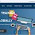## Sunday, April 21, 2013

### Matlab Program to Watermark/ Extract/ Calc PSNR / Salt Attack (Steganography)

X. Write a Matlab program that received a host image (512x512). Embed an watermark image into host image by using;
i. Embed at 8th bit then check PSNR
ii. Embed at 7th bit then check PSNR
iii. Embed at 6th bit then check PSNR
iv. Embed at 5th bit then check PSNR

Test robustness by using salt and pepper with level 0.01 to 0.09.
Write another program to extract the watermark after the watermarked image has been attacked. Display the all the images produced.

For getting the best result PLEASE TRY ON GRAYSCALE BITMAP IMAGES !!!

Water Marking Program
clc;
bit=input('Please enter which bit you want to use for watermarking? (8 | 7 | 6 | 5)');
[filename1,pathname]=uigetfile('*.*','Please select the cover image');
'www.root25.com developed by Amir
figure(1);
imshow(img1);
[row,col]=size(img1)
area=row*col;
i=1;
j=1;
k=1;
[filename2,pathname]=uigetfile('*.*','Please select the watermark image');
[w1,w2] = size(water_img);
imshow(water_img);
wm=dec2bin(water_img);
Wlength=w1*w2*8;  %
host=dec2bin(img1);
cnt=0;
while i < area
cnt=cnt+1;
if cnt>Wlength
break;
end
host(i,bit)=wm(j,k);
k=k+1;
if k>8
k=1;
j=j+1;
end
i=i+1;
end
key1=w1
key2=w2

final=bin2dec(host);
final=reshape(final,row,col);
img1(1:row,1:col)=final(1:row,1:col);
output_filename=[num2str(bit), 'th_bit_watermarked.bmp'];
imwrite(img1,output_filename);
imshow(img1);

PSNR Calculator Program

clc;
[filename1,pathname]=uigetfile('*.*','Please select the original image');
'www.root25.com developed by Amir
[filename2,pathname]=uigetfile('*.*','Please select the watermarked image');
figure(1);
imshow(original);
figure(2);
imshow(watermarked);
[row,col] = size(original)
size_host = row*col;
o_double = double(original);
w_double = double(watermarked);
s=0;
for j = 1:size_host;
s = s+(w_double(j) - o_double(j))^2 ;
end
vr=s/size_host;
psnr =10*log10((255)^2/vr);
display 'The PSNR is :',psnr

Salt & Pepper Attack Program
clc;
[filename1,pathname]=uigetfile('*.*','Please select the watermarked image');
'www.root25.com developed by Amir
Attack_watermarked=imnoise(watermarked,'salt & pepper',0.09);
% this number will change between 0.01 to 0.09
imwrite(Attack_watermarked,strcat('salt_09_',filename1));

Extraction Program
clc;
bit=input('Please define whic bit you want to extract from? (8 | 7 | 6 | 5)');
[filename1,pathname]=uigetfile('*.*','select the image');
imshow(img1);

'www.root25.com developed by Amir

[row,col]=size(img1);
Hlength=row*col;
i=1;
j=1;
k=1;
row=150; % our embed row
col=150; % our embed col
wmimage=imresize(wmimage,[row col]);
wm=dec2bin(wmimage);
Wlength=row*col*8;
host=dec2bin(img1);
cnt=0;
while i <= Hlength
cnt=cnt+1;
if cnt>Wlength
break;
end
wm(j,k)=host(i,bit);
k=k+1;
if k>8
k=1;
j=j+1;
end
i=i+1;
end

wm1=bin2dec(wm);
wm2=reshape(wm1,row,col);
wmimage(1:row,1:col)=wm2(1:row,1:col);
output_filename=['extract_', filename1];
imwrite(wmimage,output_filename);
imshow(wmimage);

Watermarking Result

Extraction Result

We can conclude from the result of the watermark that the best result is when we try to embed using the 8th bit least significant bit (LSB) and when we used the 5th bit we got the worse result and clearly we can see by eyes that something is wrong with image.

1.The content is well recognized, so no one could claim that it is just one person's opinion yet it covers and justifies all the valid points. Hope to read some more work from you.
Data Science training in Mumbai
Data Science course in Mumbai
SAP training in Mumbai

2.3.4.5.